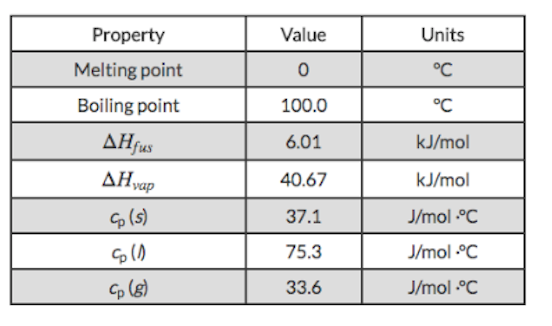# Problem: Based on the thermodynamic properties provided for water, determine the energy change when the temperature of 0.850 kg of water decreased from 123°C to 19.0°C.

🤓 Based on our data, we think this question is relevant for Professor Cheng's class at UCR.

###### FREE Expert Solution

We’re being asked to calculate the amount of energy released when 0.850 kg of water is cooled from 123 ˚C to 19.0 ˚C.

Note that cooling and freezing is an exothermic process, which means q is negative (–)

There are two heats involved in this problem:

1. q1 which is the heat in cooling 0.850 kg of H2O from 123 ˚C to 100 ˚C

2. q2 which is the heat in condensing 0.850 kg of H2O at 100 ˚C

2. q3 which is the heat in cooling 0.850 kg of H2O from 100 ˚C to 19.0 ˚C

We need to solve for each heat individually then add them together to get the final answer.###### Problem Details

Based on the thermodynamic properties provided for water, determine the energy change when the temperature of 0.850 kg of water decreased from 123°C to 19.0°C.